# TARGET SBI EXAM 2018: QUANTITATIVE- Simplification

Dear Bankersdaily Aspirants,

Hope , Your preparation for SBI Clerk & PO exam 2018 is good . Since SBI Clerk & PO exam is a Two level which will be conducted in an online mode, Competition will be higher for this SBI Clerk & PO Exam and the most important thing , Current affairs section will be there in this exam. So aspirants have to concentrate in Four sections namely Aptitude, Reasoning, English, Current Affairs.

The person with high speed and very good accuracy only can crack this exam to taste the success. The candidates will be selected on the basis of performance in this online exam test. So the candidate with consistent hard work and regular practice will crack the exam very easily.

Exam: SBI  Clerk & PO Exam 2018

Topic: Simplification

Timing: 7 minutes

What value should come in place of question mark (?) in the following equations?

Q.1) 21.12+1221.21-21.21+12.21+21.01=?

(a) 1245.34

(b) 1254.34

(c) 1134.76

(d) 1142.43

(e) None of these

What value should come in place of question mark (?) in the following equations?

Q.2)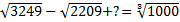(a) 10

(b) 90

(c) 5

(d) 0

(e) 17

What value should come in place of question mark (?) in the following equations?

Q.3)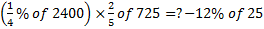(a) 1765

(b) 1868

(c) 1439

(d) 1743

(e) None of these

What value should come in place of question mark (?) in the following equations?

Q.4) (81)3 × 98 ÷ (729)3 = 9?

(a) 5

(b) 0

(c) 12

(d) 7

(e) None of these

What value should come in place of question mark (?) in the following equations?

Q.5) 1.2×1.3×1.4×1.5×?=39.312

(a) 13

(b) 22

(c) 12

(d) 27

(e) None of these

What value should come in place of “x” in the following questions?

Q.6)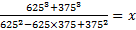(a) 1000

(b) 1024

(c) 1225

(d) 1331

(e) None of these

What value should come in place of “x” in the following questions?

Q.7) 4096 × 8= 8× 8÷ 512

(a) 1

(b) 2

(c) 3

(d) 4

(e) None of these

What value should come in place of “x” in the following questions?

Q.8) 1=1x

(a) 0

(b) 1

(c) 2

(d)  Can’t be determined

(e) None of these

What value should come in place of “x” in the following questions?

Q.9)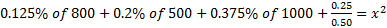(a) 2.5

(b) 3.5

(c) 4.5

(d) 5.5

(e) 8

What value should come in place of “x” in the following questions?

Q.10) 0.25×25-1+6.75+22+32=x2

(a) 6

(b) 5

(c) 7

(d) 8

(e) 9

You can check the explanations from the one that is given below. If you have scored well in this, then your preparations is in the right direction.

Explanation

Q.1)

b

21.12+1221.21-21.21+12.21+21.01=?

1275.55-21.21=1254.34

Q.2)

d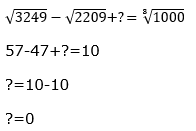Q.3)

d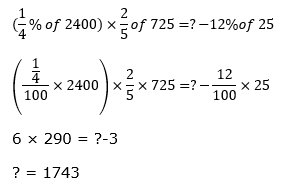Q.4)

a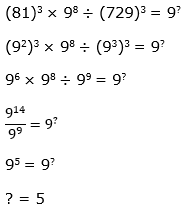Q.5)

c

1.2×1.3×1.4×1.5×?=39.312

3.276×?=39.312

?=12

Q.6)

a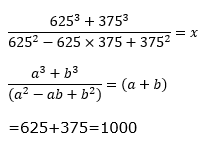Q.7)

e

8x=8(4+3-3-4)

8x=80

x=0

Q.8)

d

11=1x =>x=1 and 10=1x   =>x=0……etc.

So, can’t be determined.

Q.9)

a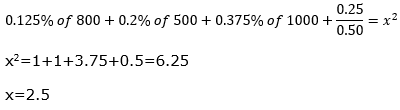Q.10)

b

0.25×25-1+6.75+22+32=x2

x2=6.25-1+6.75+4+9=25

x=5